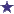# Calculating daily maintenance fluids for pediatrics

by Sherri
(Raleigh NC)I am stuck on the 100/50/20 procedure. Can you walk me through these to see how my book got its answers?

1. Total volume and hourly IV flow rate for a 3500g infant receiving maintenance fluids. Infuse____ ml at ____ml/hr. They have 2 and 23.Do I calculate it as 3500 g or 3.5 kg? what do I put for the 100, 50, and 20?

2. Total volume and hourly IV flow rate for a 13 kg child receiving maintenance IV fluids. Infuse ___ml at ____ml/hr. They have 80 and 6. How do they get this and what do I put for 100/50/20?

4. Total volume and hourly IV flow rate for a 25 kg child receiving maintenance IV fluids. Infuse ____
ml at____ ml/hr. They have 120 and 3.How do they get this and what do I put for 100, 50, and 20?

6. Total volume and hourly IV flow rate for an 8800g child receiving maintenance IV fluids. Infuse___ ml at ____ml/hr. Of course this is like #1.They have 880 and 35 for their answers. Again, how do they get this and what do I put for 100, 50, and 20?

Average RatingRatingPediatric IV Math For Fluid Administration by: Anonymous The rule for calculating maintenance fluids for a 24 hours period, is the 100/50/20 rule. IV administration of 100ml/kg for the first 10kg is given. Then 50ml/kg for the next 10kg and then 20ml/kg for every kg over 20. Once this has been figured, you divide the amount by 24 to get the hourly IV fluid flow rate. Being able to calculate pediatric IV math when working with children is very important. All facilities have policies and protocols that are to be followed for the calculations and administration of the pediatric fluids.The following table of fluid requirements may be used to help:Weight range Required Daily Fluid0-10 kg 100ml per kg10-20kg 1,000ml +50ml per each kg above 10kg20-70 kg 1,500ml +20ml per each kg above 20kgOver 70kg 2,500ml (adult requirement) When preparing to calculate the IV fluid amounts that are needed for the patient, calculate the weight in kg for the formula. To calculate the hourly maintenance fluid requirements for a child weighing 5kg:100ml x 5kg =500ml/24 hours500ml divided by 24 hours= 20.8 ml/hrIn order to figure the hourly maintenance fluid requirements for a child that weighs 3.5kg:100ml x 3.5kg =350ml/24 hours350ml divided by 24 hours=14.5ml/hrTo calculate the hourly maintenance fluid requirements for a child weighing 13kg:1,000ml (which is the 100ml x 10kg) + 150ml (which is the 50ml x each kg over the 10kg) =1,150ml/24 hours1,150ml divided by 24 hours = 47.9ml/hrTo calculate the hourly maintenance fluid requirements for a child weighing 25kg:1,500ml (which is the 100ml x 10kg + 50ml x each kg over 10kg) + 100ml (20ml x each kg over 20 kg) = 1,600ml/24 hours1,600ml divided by 24 hours = 66.6ml/hrTo Calculate the hourly maintenance fluid requirements for a child weighing 8.8kg:100ml x 8.8kg = 880 ml/24hours880ml divided by 24hrs =36.6ml/hr Calculating IV Rates: Drops per minute Total volume to be given (in ml's) Drop factor____________________________ X ______________ = Drops per min.time in minutes 1 The calculation of pediatric fluids and dosages is of the upmost importance and can easily be rechecked by calling the pharmacists or pharmacy technician, to double check your calculations, prior to administration.